## 0. Preparation

In :
``````%matplotlib inline

#Load packages
import numpy as np
import pandas as pd
import os
import pickle
import datetime
from scipy import stats
from statsmodels.tsa.stattools import pacf
import matplotlib.pyplot as plt
import matplotlib.dates as mdates
import pylab``````
In :
``````#Import time series
data = pd.read_pickle('FS2019.pkl')``````

## 1. Statistical modelling of past observations

#### 1.a)

In :
``time = np.arange(0, len(data), 1)``
In :
``````model = stats.linregress(time, data.values)
fig = plt.plot(time, data.values)
plt.figure()
fig = plt.plot(data)
``````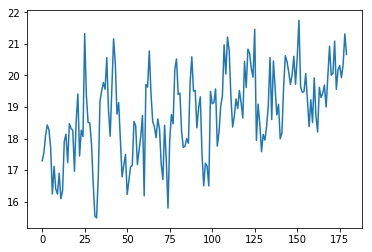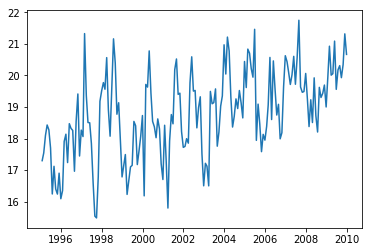In :
``model``
Out:
``LinregressResult(slope=0.013894661458922097, intercept=17.549236987549193, rvalue=0.5364361003700527, pvalue=8.40168729286144e-15, stderr=0.0016384456024656614)``
In :
``````a = model.slope*time + model.intercept
plt.plot(time, data.values)
plt.plot(time, a)

percent = (len(time)*model.slope)/data.values
print('The values increase by', percent, '% over the covered time period.')``````
```The values increase by 0.1445901596577515 % over the covered time period. ```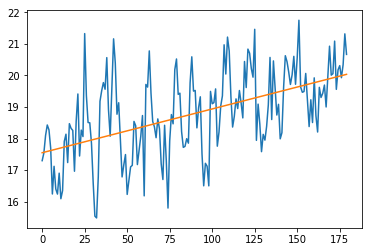What is the corresponding trend estimate 𝛽0 and 𝛽1?

𝛽0 = 17.54924 m/s 𝛽1 = 0.01389 (m/s)/month

#### 1.b)

What other method can be applied to estimate the trend?

• fitting a higher polynomial or exponential
• different methods of moving averages (moving average, exponential weighted moving average, ...)
• filters (median filters, bandpass filters, ...)

#### 1.c)

Is the trend significant on a 5 % level?

???

#### 1.d)

In :
``````residuals = data.values - a
plt.plot(time, residuals)
length = len(time)
zeros = np.zeros((length,1))
fig = plt.plot(time, zeros)
``````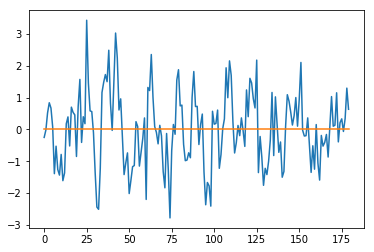What are the iid assumptions on the residuals for the linear regression and are they fulfilled? Discuss each assumption with a plot and 1-2 sentences using the detrended data.

iid assumptions: independent and identically normally distributed

independent:

In :
``````autocorrelation = pacf(data.values, nlags=20, method='yw')
x = np.arange(0, len(autocorrelation), 1)

plt.stem(x, autocorrelation)
# plot_pacf(data.values, lags=20)
ax = plt.xticks(np.arange(0, len(autocorrelation), 1))

plt.plot(np.arange(0, len(autocorrelation), 1), np.full((len(autocorrelation),), 0.2), 'r:')
plt.plot(np.arange(0, len(autocorrelation), 1), np.full((len(autocorrelation),), -0.2), 'r:')

``````
Out:
``[<matplotlib.lines.Line2D at 0x1c1c4bf0b8>]``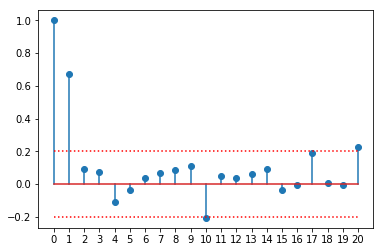The partial autocorrelation plot shows a significant correlation between the time series values and its predecessor with lag-1. Values with bigger lag are not longer clearly correlated. This indicates an autoregressive term of order 1.

identically normally distributed:

In :
``````plt.hist(residuals, bins=15, density=True, stacked=True)

x = np.linspace(stats.norm.ppf(0.01), stats.norm.ppf(0.99), 100)
plt.plot(x, stats.norm.pdf(x),'r-', lw=5, alpha=0.6, label='normal pdf')
plt.legend(loc='best', frameon=False)

``````
Out:
``<matplotlib.legend.Legend at 0x1c1c505e80>``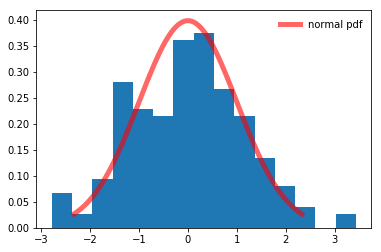In :
``````fig = plt.figure()
probplot = stats.probplot(residuals, dist=stats.norm, plot=plt)
``````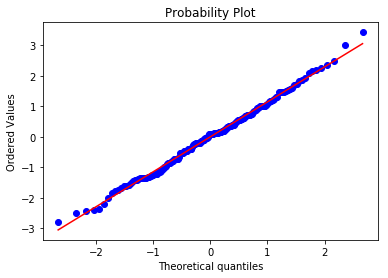The histogramm of the residuals shows a decent overlapping with the theoretical normal distribution. This is confirmed in the quantile-quantile-plot that shows that the quantiles of the residuals versus the theoretical quantiles of the normal distribution. They lie almost exclusively on the diagonal, indicating that the residuals don't differ much from a normal distribution.

#### 1.e)

Can you come up with physical explanations for the outcome of 1.d)? Write maximal two sentences.

???

#### 1.f)

What is the order (p) of the partial auto-correlation of the noise in your time series? Look at the plot you created in 1.d) using pacf() for this purpose. What is the partial auto-correlation 𝛼 of the lag-1?

In :
``````alpha = autocorrelation
print(alpha)
``````
```0.6706273043878814 ```

The pacf-plot suggests an partial autocorrelation of order 1. The partial autocorrelation of lag-1 is: 0.67063

#### 1.g)

How does the autocorrelation affect the trend estimate (1.a)) and variance? What does this imply for the significance of the trend you have estimated in 1.c)?

???

#### 1.h)

*What is the standard deviation (σ) of 𝑤 assuming a normal distribution? Remember that 𝑤 = 𝜖(t)𝛼 − 𝜖(t+1) according to equation 2.

In :
``````length = len(residuals)
w = np.zeros((length,1))
for i in range(length-1):
w[i] = residuals[i]*alpha - residuals[i+1]

sigma = np.std(w)
print(sigma)
``````
```0.9632893692409062 ```

The standard deviation of w is 0.96329.

#### 1.i)

Reproduce the observations by generating an additional realization. Does your model reproduce the characteristics of the observations?

In :
``````realised_stochastic = np.random.normal(0, sigma, len(data.values))

realised_residuals = np.zeros((len(data.values),1))
realisation = np.zeros((len(data.values),1))

for i in range(1,len(data.values)):
realised_residuals[i] = realised_residuals[i-1]*alpha + realised_stochastic[i-1]

for i in range(len(data.values)):
realisation[i] = model.intercept + model.slope*time[i] + realised_residuals[i]

#plt.plot(time, realised_stochastic)
#plt.plot(time, realised_residuals)
#plt.figure()

fig = plt.plot(time, data.values)
fig = plt.plot(time, realisation)
fig = plt.plot(time, a)

``````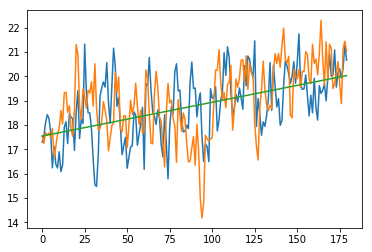first term of residuals on regression line?!

Yes, the realisation of the model reproduces the observations quite well.

## 2. Probabilistic realizations of the historical and a future time period

#### 2.a)

After conducting the Monte-Carlo simulation with 10'000 realizations, plot all the forecasts together with the historical time series and the estimated trend in one figure to get a feeling of the spread of SI.

In :
``````def monte_carlo_SI(y, b0, b1, alpha, sigma, n):
x = np.arange(0,len(y), 1)
realisation = np.zeros((len(y),n))

for j in range(n):
wt = np.random.normal(0, sigma, len(y))
et = np.zeros((len(y),1))

for i in range(1,len(y)):
et[i] = et[i-1]*alpha + wt[i-1]

for i in range(len(y)):
realisation[i,j] = b0 + b1*x[i] + et[i]

return realisation

n = 10000``````
In :
``````all_realisations = monte_carlo_SI(data.values, model.intercept, model.slope, alpha, sigma, n)
fig = plt.plot(time, all_realisations, 'r-', linewidth = 0.1)
# fig = plt.plot(time, a, '#ff7f0e', linewidth = 2)
fig = plt.plot(time, a, 'k:', linewidth = 2)
fig = plt.plot(time, data.values, 'k-', linewidth = 3)``````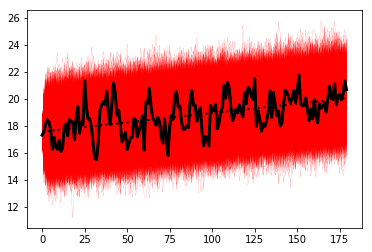#### 2.b)

Plot the median of all realizations for each month. With the function plt.fill_between() you can plot area between (1) the interquartile range and (2) the 2.5 and 97.5 percentile and (3) the minimum and maximum value per month. Use different transparency for the different ranges controlled by the function argument alpha.

In :
``````all_medians = np.zeros((len(data.values),1))
all_medians2 = np.zeros((n,1))
all_25 = np.zeros((len(data.values),1))
all_75 = np.zeros((len(data.values),1))
all_2dot5 = np.zeros((len(data.values),1))
all_97dot5 = np.zeros((len(data.values),1))
all_max = np.zeros((len(data.values),1))
all_min = np.zeros((len(data.values),1))

for i in range(len(data.values)):
all_medians[i] = np.median(all_realisations[i,:])
all_25[i] = np.percentile(all_realisations[i,:], 25)
all_75[i] = np.percentile(all_realisations[i,:], 75)
all_2dot5[i] = np.percentile(all_realisations[i,:], 2.5)
all_97dot5[i] = np.percentile(all_realisations[i,:], 97.5)
all_max[i] = np.percentile(all_realisations[i,:], 100)
all_min[i] = np.percentile(all_realisations[i,:], 0)    ``````
In :
``````x = np.arange(0,len(data.values), 1)
fig = plt.fill_between(x, all_max[:,0], all_min[:,0], alpha = 0.2, color = 'r', linestyle = '-', linewidth = 0.5)
fig = plt.fill_between(x, all_97dot5[:,0], all_2dot5[:,0], alpha = 0.4, color = 'r', linestyle = '-',linewidth = 0.5)
fig = plt.fill_between(x, all_75[:,0], all_25[:,0], alpha = 1, color = 'r', linestyle = '-', linewidth = 0.5)
fig = plt.plot(x, all_medians, 'k-', linewidth = 1)
fig = plt.plot(x, data.values, 'b:', linewidth = 1)

plt.savefig('bandwidth.png')``````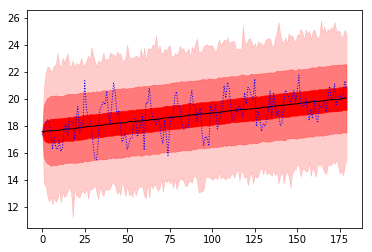## 3. Forecast and “Reality” – a little excursus

In [ ]:
`` ``